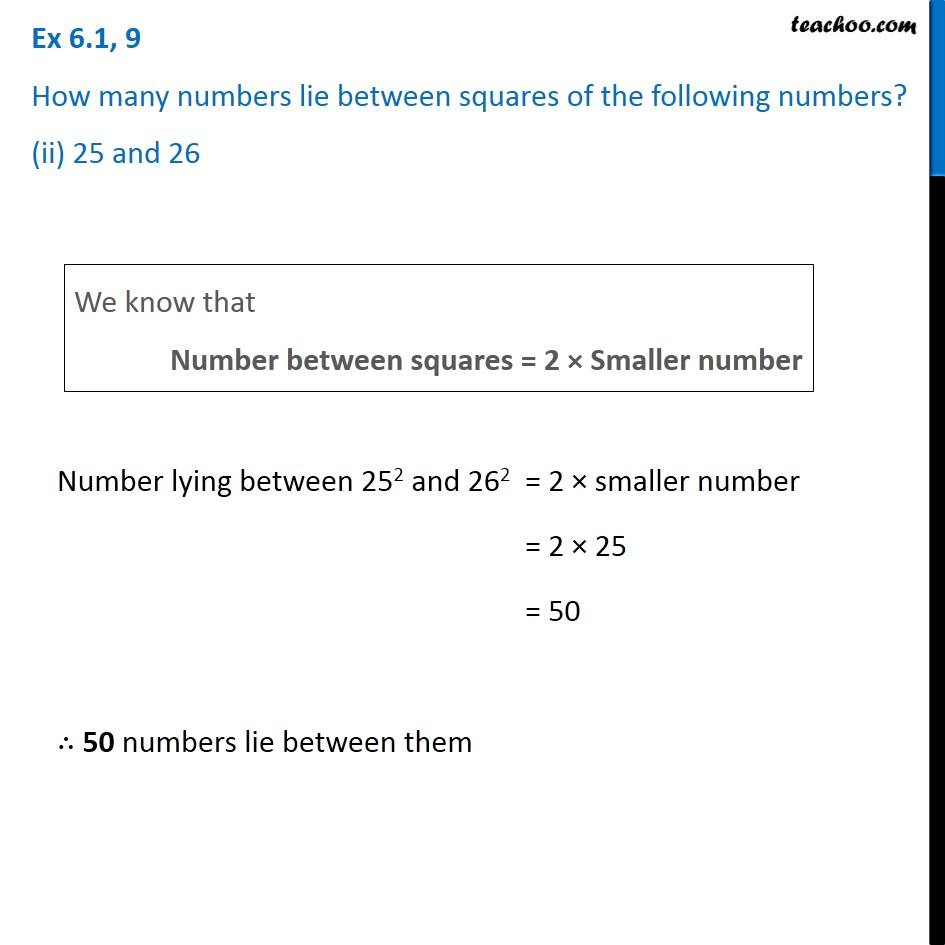Ex 6.1

Chapter 6 Class 8 Squares and Square Roots
Serial order wiseGet live Maths 1-on-1 Classs - Class 6 to 12

### Transcript

Ex 6.1, 9 How many numbers lie between squares of the following numbers? (ii) 25 and 26We know that Number between squares = 2 × Smaller number Number lying between 252 and 262 = 2 × smaller number = 2 × 25 = 50 ∴ 50 numbers lie between them